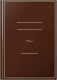Books BooksIf two right-angled triangles have their hypotenuses equal, and one side of the one equal to one side of the other, the triangles are congruent.Euclid's Elements of Geometry: From the Latin Translation of Commandine. To ... - Page 231
by Euclid, John Keill - 1723 - 364 pages## Calendar

University of Birmingham - 1904 - 588 pages## A Shorter Geometry

462 pages
...equal, (p. 56.) FACT N [Th. I. 15]. If two right.angled triangles have their hypotenuses equal, and one side of the one equal to one side of the other, the triangles are congruent, (p. 59.) All references to the above will, in future, be to the number...## The calendar of the University college of Wales

Wales univ, univ. coll. of Wales - 1878 - 188 pages
...treatise. 19. Solve 3 . ( ' ~ x + 2a VII.— GEOMETRY. EUCLID I., II., III. 1. If two triangles have one side of the one equal to one side of the other, and the angles at the extremities of those sides equal, each to each, the triangles are equal in all...## The New Geometry: Form One

G. P. West - Geometry - 1965 - 370 pages
...QED THEOREM 13. If two triangles have one angle in each a right angle, their hypotenuses equal, and one side of the one equal to one side of the other, the triangles are congruent. 6,r Given. RST, XYZ are two triangles which have ZT and ZZ each a right...## Calendar

University of Cambridge - 1913 - 1632 pages## Examinations Papers

1876## Paractical Geometry Based on the Various Geometry Books by Godfrey and Siddons

480 pages
...should be consulted. PAGE THEOREM 15. If two right-angled triangles have their hypotenuses equal, and one side of the one equal to one side of the other, the triangles are congruent 24 NOTE ON THE AMBIGUOUS CASE 24 EXERCISES 25 Chapter IV. CONSTRUCTIONS...## The New Zealand University Calendar, Volume 32

University of New Zealand - 1904 - 360 pages
...each, the triangles are congruent. If two right-angled triangles have their hypotenuses equal, and one side of the one equal to one side of the other, the triangles are congruent. If two sides of a triangle are unequal, the greater side has the greater...## Geometry for Beginners

100 pages
...equal- (p. 56.) FACT N [Th. I. 15]. If two right-angled triangles have their hypotenuses equal, and one side of the one equal to one side of the other, the triangles are congruent- (p. 59.) Geometry for Beginners 1-00-136893-2 €01 368931 ...